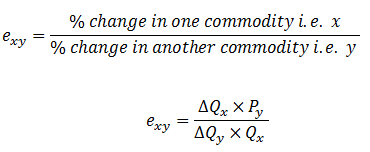# Cross elasticity of demand

It refers the percentage change in quantity demand of one commodity i.e. x due to certain change in price of another commodity i.e. y when other things remains the same.

Mathematically,where,
exy = cross elasticity of demand
∆Qx = change in quantity demand for x commodity
∆Py = change in price of y commodity
Py = initial price of y commodity
Qx = initial quantity demand for x commodity